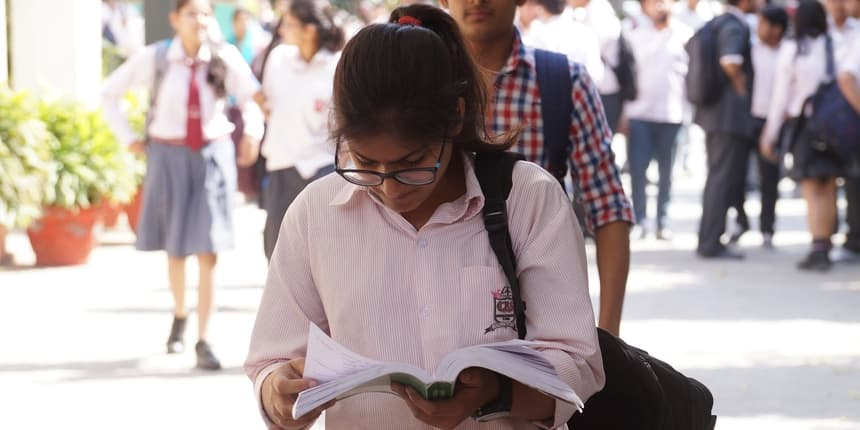# CBSE Class 10 maths exam today; Marking scheme, deleted syllabus, chapter-wise weightage

## Candidates can check the CBSE Class 10 chapter-wise weightage, blueprint, deleted syllabus and marking scheme here.CBSE Class 10 maths. (Picture: Shutterstock)
Ishita Ranganath | Mar 21, 2023 - 7:07 a.m. IST
Share Via

NEW DELHI: The Central Board of Secondary Education (CBSE) will be conducting the CBSE Class 10 mathematics paper today. Candidates can check the CBSE Class 10 chapter-wise weightage, blueprint and marking scheme here. Students can find the Class 10 sample papers 2023 on CBE's official website- cabseacademic.nic.in.

CBSE Class 10 students will be appearing for their last board exam 2023 today. The maths exam will be held from 10:30 AM to 1:30 PM. Candidates will not be allowed to carry any electronic devices or calculators to the exam hall.

Also Read | CBSE to implement NCF for foundation stage from 2023-24 academic session

## CBSE Class 10 maths deleted syllabus 2022-23

CBSE Class 10 Maths Syllabus 2022-23 is reduced by 30% and the topics removed will not be assessed in the upcoming CBSE Board Exam 2023.

 Chapter Topics Real Numbers Euclid’s division lemma, Decimal representation of rational numbers in terms of terminating/non-terminating recurring decimals. Polynomials Statement and simple problems on division algorithm for polynomials with real coefficients. Pair of linear equations in two variables Simple problems on equations reducible to linear equations. Coordinate geometry Area of a triangle Triangles Proof of the following theorems are deleted If a perpendicular is drawn from the vertex of the right angle of a right triangle to the hypotenuse, the triangles on each side of the perpendicular are similar to the whole triangle and to each other.The ratio of the areas of two similar triangles is equal to the ratio of the squares of their corresponding sides.In a right triangle, the square on the hypotenuse is equal to the sum of the squares on the other two sides.In a triangle, if the square on one side is equal to sum of the squares on the other two sides, the angles opposite to the first side is a right angle. Constructions Full Chapter Deleted Trigonometric identities Trigonometric ratios of complementary angles Surface area and volumes Frustum of a cone.Problems involving converting one type of metallic solid into another and other mixed problems. (Problems with combination of not more than two different solids be taken). Statistics · Step deviation Method for finding the mean· Cumulative Frequency graph

## CBSE Class 10 maths blueprint 2023

The CBSE Class 10 maths theory paper will be for 80 marks, while the internal assessment will be for 20 marks. The exam will consist of 30 questions divided into four sections with 1, 2, 3, and 4 mark questions. The duration of the paper is three hours.

SectionNo. of questionsTotal marks
A: MCQs
(1 mark)
2020
B: SA-I
(2 marks)
510
C: SA-II
(3 marks)
618
D: LA
(5 marks)
420
E: CB
(4 marks)
312
Grand total38 questions80 marks